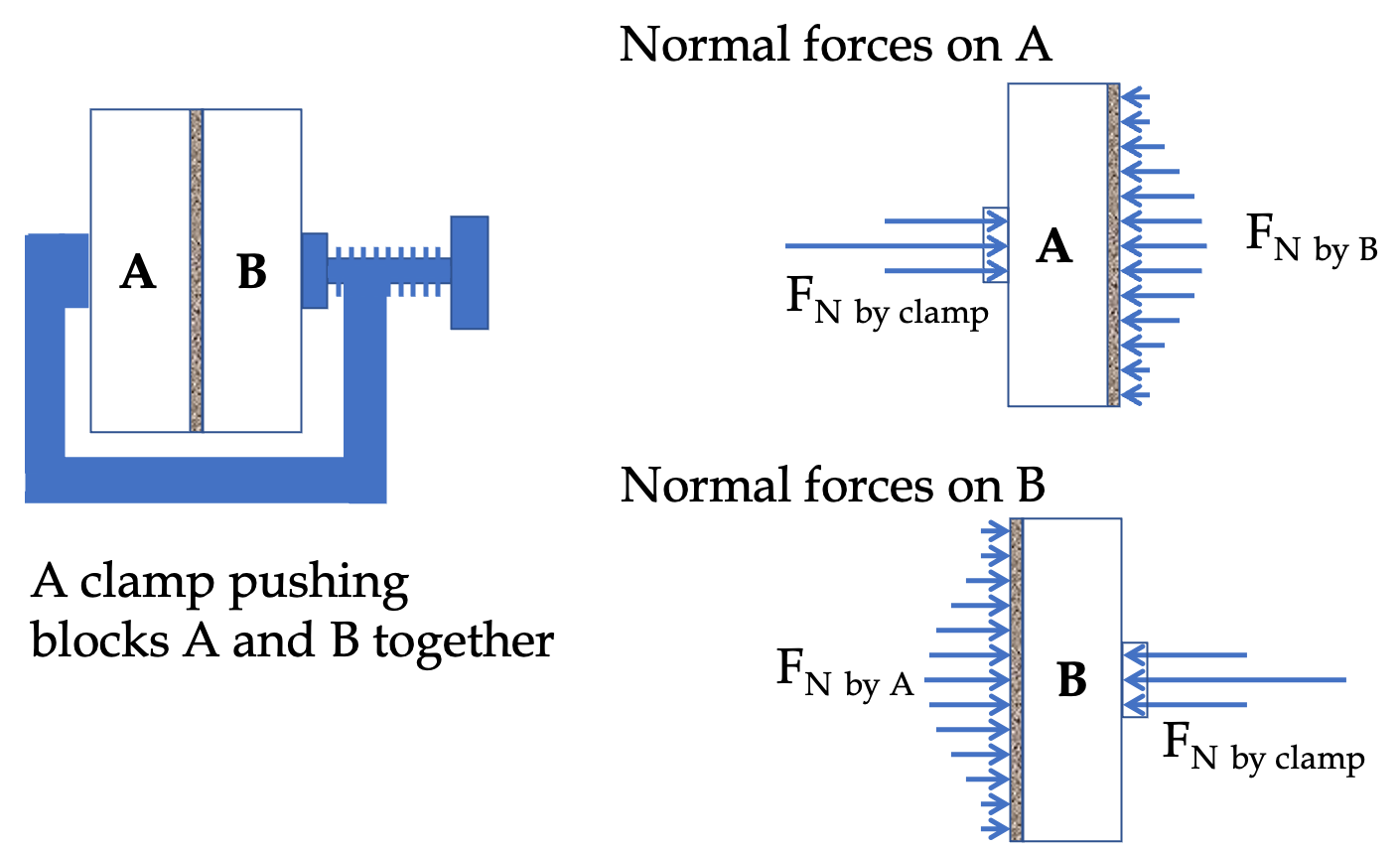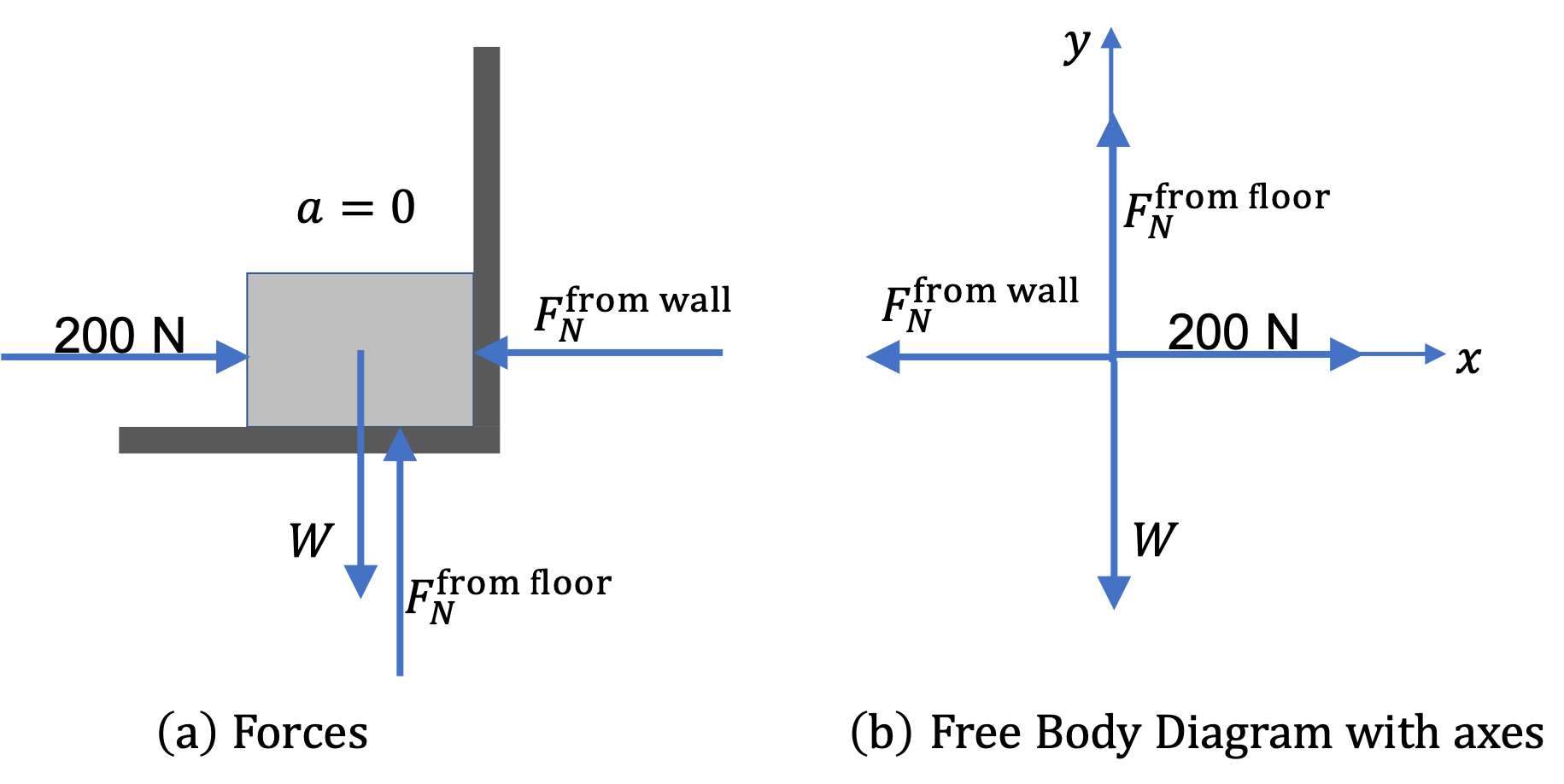## Section6.8Normal Force

When two solid bodies are pushed into each other, they develop a repulsive force at the surface (or point) of contact. Why does that happen? Think of molecules of each solid body as springs, which become compressed when pushed in from outside. As a result, they push out and away from the body. This reactive force is called the normal force since its direction is perpendicular to the surface of contact.

We will denote normal force by either symbol $\vec F_N$ or $\vec N \text{,}$ and the magnitude by the same symbol without an arrow over the symbol. You can see confusion arizing from the use of $\vec N\text{,}$ its magnitude symbol will be $N\text{,}$ which is same symbol as the unit of force. For instance, in $N=50\text{ N}\text{,}$ the first $N$ is the name Normal and the second is the unit $\text{N}\text{.}$Figure 6.8.1. When you push two blocks into each other by a clamp, normal forces develop at all surfaces of contact as shown in thid figure.

Unlike the force of weight, there is no formula for the magnitude of the normal force. If you have memorized some formula for the magnitude of the normal force, such as $F_N = mg$ and $F_N = mg\cos\,\theta\text{,}$ then you need to forget that incorrect knowledge. You cannot get the magnitude of the normal force without applying Newton's second law of motion.

As far as the direction is concerned, we have the guidance that the force is repulsive. Therefore, for a normal force between two bodies A and B, when the normal force acts on A, it is pointed away from B, and when it acts on B, it is pointed away from A.

Normal force is perpendicular to the surface of contact. It is easy to get the normal direction if the surface of contact is planar. If the surface of contact is curved, the direction of normal force will vary over the surface of contact, being normal to the local tangent plane of the surface.

Normal force across a surface of contact may not have the same value. It will be greater where the bodies are more pressed into each other and less where less pressed as illustrated in Figure 6.8.1 and Figure 6.8.2.

The box on the wedge presses more at points that are at lower points since the weight of more parts of the box acts there. This results in more normal force in lower parts than upper parts. That means the net normal force does not go through the center of the box, but a little bit right of the center in this figure.

Therefore, when we draw a single arrow to represent normal force on a body, we let the arrow pass through the center of the surface of the contact if normal force is uniform across the surface, otherwise we draw the arrow more near where the normal force is expected to be larger.

A 50-kg box is pushed against a wall by a force that acts horizontally and has magnitude $200\text{ N}\text{.}$ Ignore any friction forces for this problem. What are the magnitudes and directions of all forces on the box?

Hint

Think also a force from the wall.

$N_w = 200\text{ N},\ \ N_f=490.5\text{ N}.$

Solution

System: the 50-kg box.

Identifying forces on the system:

We first try to identify forces on the box. Usually a diagrram such as shown in Fig. (a) helps. Force on the box will come from other objects in the environment, either through a contact with them or through non-contact forces, such as gravity.

The objects in the environment are: Agent doing the pushing, Earth, Floor, and Wall. Each of them will apply force(s) on the box. Assuming no friction forces, we identify the following forces.

1. Push, $\vec F \text{,}$
2. Force of weight by Earth, $\vec W\text{,}$
3. Normal Force from the floor, $\vec F_N^{\text{from floor}}\text{,}$
4. Normal Force from the wall, $\vec F_N^{\text{from wall}}\text{.}$

If we were not given the instruction that friction force could be ignored, we would have two more forces.

1. Static Friction Force from the floor, $\vec F_s^{\text{from floor}} = 0\text{,}$
2. Static Friction Force from the floor, $\vec F_s^{\text{from wall}} = 0\text{.}$Figure 6.8.5. (a) Sketch of forces on the box along with the box, floor, and wall. (b) Redrawing the forces in a simpler figure of just the forces in the free-body diagram. Choice of axes is also shown in (b)

The Free-Body Diagram:

It is almost always very helpful to strip away the details and redraw a sketch of just the forces as in Figure 6.8.5(b). In this simplified picture, called the free-body diagram, we draw the force vectors with tails of all forces placed at the same point. We take the point where tails are located as the origin of a Cartesian coordinate system.

Choosing Cartesian Axes:

In this step, if we expect acceleration, then it is almost always helpful to point one of the Cartesian axes in the direction of the acceleration. But, if the acceleration is zero, or the direction of acceleration is unknown, then we prefer to draw axes that would simplify the computation of net force, which happens when most of the forces are along the axes.

Here, the acceleration is zero. Furthermore, we notice in Fig. Figure 6.8.5(a) that if we use axes horizontally and vertically, every force will be along the Cartesian axes. This will make computation of components of $\vec F_{\text{net}}$ easier.

Generating equations of motion:

Since $\vec a = 0 \text{,}$ our equation of motion is:

\begin{equation*} \vec F_{\text{net}} = 0, \end{equation*}

which would result in two equations, one along each Cartesian direction.

Although not necessary, sometimes it is helpful to collect the components in a table form. Let us use simpler symbols $N_f$ and $N_w$ for the magnitudes of the normal forces from the floor and from the wall respectively.

The $x$ component of the net force will be just the sum of the $x$ components of all the forces. Similarly for the $y$ component of the net force.

\begin{align*} \amp F_{x}^{\text{net}} = 0 + 200 + 0 - N_w,\\ \amp F_{y}^{\text{net}} = -490.5 + 0 + N_f + 0. \end{align*}

Since $\vec F_{\text{net}} = 0\text{,}$ we get the following two equations.

\begin{align*} \amp 0 + 200 + 0 - N_w = 0\\ \amp -490.5 + 0 + N_f + 0 = 0. \end{align*}

Solve for the desired quantities:

This turns out to be easy here since each of the two equations of motion has only one unknown.

\begin{equation*} N_w = 200\text{ N},\ \ N_f=490.5\text{ N}. \end{equation*}

Interpret the result:

We have found the magnitudes of the two normal forces on the box. The normal force from the floor has magnitude $490.5\text{ N}$ and pointed up, and the normal force from the wall has magnitude $200\text{ N}$ and pointed away from the wall and perpendicular to the wall.

A book of unknown mass can be supported against a vertical frictionless wall by applying a $10\text{ N}$-force at an angle $60^{\circ}$ with the horizontal direction. Note: three forces are acting on the book.

(a) Draw a free-body diagram showing with standard symbols and arrows all the forces acting on the book.

(b) Find the magnitude and direction of weight and normal force on the book.

Hint

Use $\vec F_{\text{net}} = 0\text{.}$

(a) See solution (a), (b) $F_N = 5\text{ N}\text{,}$ $W = 8.66\text{ N}\text{,}$ and the directions as shown in figure in (a).

Solution 1 (a)

(a) Figure 6.8.9 contains two panles - the one on the left helped me identify the forces, which I then simplified in the one on the right. The static friction bewteen the book and the wall is missing because we have been insrtucted in the problem statement that the wall is frictionless.

Solution 2 (b)

(b) Since the forces are balanced, the net force is zero.

\begin{equation*} \vec F_{\text{net}} = 0. \end{equation*}

The Cartesian components of this equation will give us two equations fromwhich we can get the values of the magnitudes $W$ and $N \text{.}$

\begin{align*} \amp F_{x} = 10\,\cos\,60^{\circ} = 5,\ \ F_{y} = 10\,\sin\,60^{\circ} = 8.66,\\ \amp W_x = 0, \ \ W_{y} = -W.\\ \amp F_{Nx} = -F_N, \ \ F_{Ny} = 0. \end{align*}

Therefore.

\begin{align*} \amp 5 + 0 - F_N = 0,\\ \amp 8.66 -W + 0= 0. \end{align*}

It is now trivial to get

\begin{equation*} F_N = 5\text{ N},\ \ W = 8.66\text{ N}. \end{equation*}